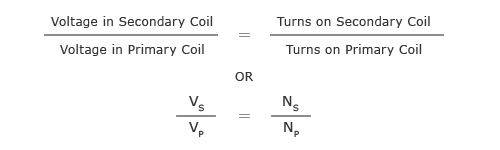## Principle of Transformers

A transformer is a device that changes (transforms) and alternating potential difference (voltage) from one value to another value be it smaller or greater using the principle of electromagnetic induction.

A transformer consists of a soft iron coil with two coils wound around it which are not connected to one another. These coils can be wound either on separate limbs of the iron core or be arranged on top of each other.

The coil to which the alternating voltage is supplied is called the primary coil or primary winding. When an alternating potential difference is supplied the resulting alternating current in the primary coil produces a changing magnetic field around it. This changing field induces an alternating current in the secondary coil. The size of the induced voltage resulting from the induced current in the secondary coil depends on the number of turns in the secondary coil.

The relationship between the voltage and the number of turns in each coil is given by:Transformers can be of two types:

## Step-up Transformer

On a step-up transformer there are more turns on the secondary coil than the primary coil. The induced voltage across the secondary coil is greater than the applied voltage across the primary coil or in other words the voltage has been “stepped-up”.

## Step-down Transformer

A step down transformer has less turns on the secondary coil that the primary coil. The induced voltage across the secondary coil is less the applied voltage across the primary coil or in other words the voltage is “stepped-down”.

Transformers are very efficient. If it is assumed that a transformer is 100% efficient (and this is a safe assumption as transformers may be up to 99% efficient) then the power in the primary coil has to be equal to the power in the secondary coil, as per the law of conservation of energy.

Power in primary coil  = Power in secondary coil

Remember, power = potential difference x current

Thus,

Primary coil p.d. x primary coil current = Secondary coil p.d. x secondary coil current

VP x IP  =  VS x IS

So if the potential difference is stepped up by a transformer then the current is stepped down by roughly the same ratio. In the case of the potential being stepped down by the transformer then the current is stepped up by the same ratio.